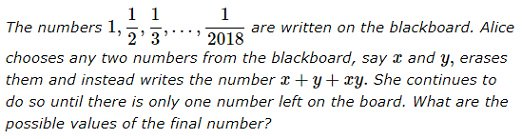## Symmetric Polynomials

This post is inspired by the video and post of Presh TalwakarCategories: Uncategorized

## Ellipses in Triangles

This post is motivated by a tweet about Steiner inellipse.The code  used:
 Module[{s = RegionCentroid[Polygon[{a, b, c}]], ab = b - a, m1, m2, m3, sc, rt}, sc = c - s; {m1, m2, m3} = Mean /@ Partition[{a, b, c, a}, 2, 1]; rt = Quiet[ ReIm[z] /. Solve[1/(z - Complex @@ a) + 1/(z - Complex @@ b) + 1/(z - Complex @@ c) == 0, z]]; ParametricPlot[s + sc Cos[t]/2 + ab Sin[t]/(2 Sqrt), {t, 0, 2 Pi}, PlotRange -> Table[{-3, 3}, 2], Frame -> True, Epilog -> {PointSize[0.03], EdgeForm[Black], FaceForm[None], Polygon[{a, b, c}], Point[{a, b, c}], Red, Point[{m1, m2, m3}], Orange, Point[s], Purple, Point[rt]}]] Manipulate[ se[p1, p2, p3], {{p1, {0, 0}}, Locator}, {{p2, {0, 1}}, Locator}, {{p3, {1, 1}}, Locator}] 

Categories: Uncategorized

## Squares

Categories: Uncategorized

## Squares and Triangles

This post is inspired by a puzzle I found on Twitter. I cannot find the source right now.  What is the area of the shaded triangle? The following gif provides the clue:Note all basic elements are squares. The small upper squares have sides of unit length. Note the whatever the triangle the base as the  upper right unit square diagonal drawn diagonal always has a height of the drawn square with side 2.

Categories: Uncategorized

## Fun with Fractions

This post is inspired by tweet from @CutTheKnotMath and his post.

He posed this puzzle for the New Year:Consider the list:$a_i, 1\le i \le n$

Consider the product:$\prod_{i=1}^n (a_i+1) -1$

For$n\ge 2$, replacing randomly chosen$a_i, a_j$ with$a_i+a_j+ a_i a_j$ in the expression does not change its value as$(a_i+1)(a_j+1)=(a_i+a_j+a_i a_j)+1$. So  repeating till there is one number allows you solve the problem, i.e. process ends a unique number. For the particular sequence this is particularly pleasing:$\prod ^n_{i=1}(1+1/i)-1=\prod^n_{i=1}\frac{i+1}{i} -1= n+1-1=n$.

Simulating a small example with Mathematica:Categories: Mathematica, Mathematics

## A Bookmark

This post is just a bookmark for a “history of cardiac catheterization”.

Categories: Uncategorized

## Fun Animation